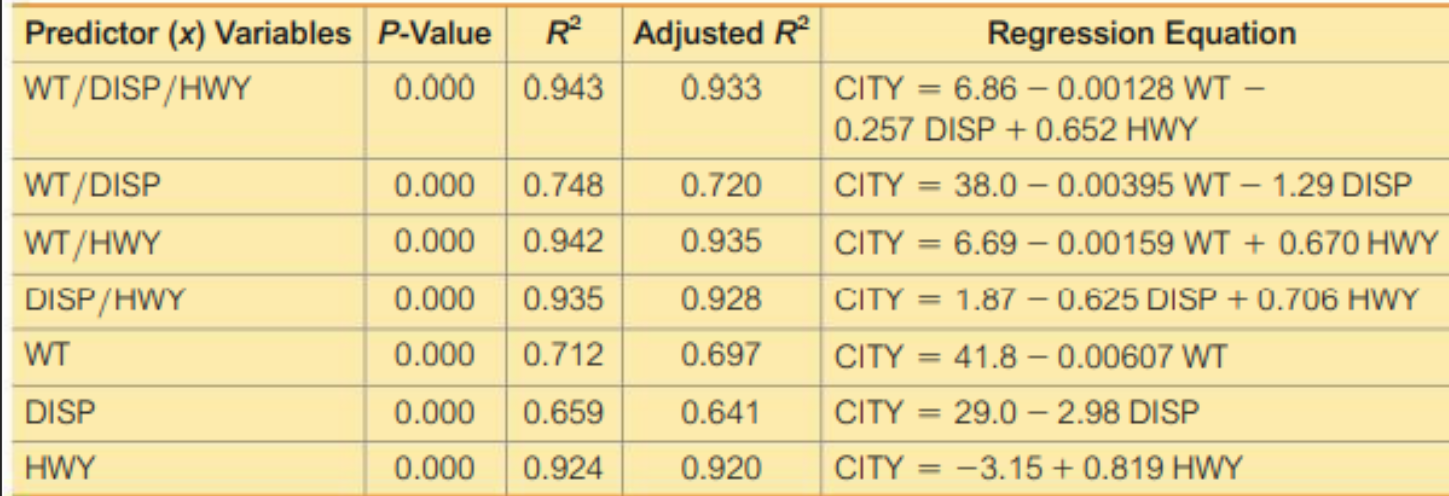### Create an Account

Home / Questions / Which regression equation is best for predicting city fuel consumption Why

# Which regression equation is best for predicting city fuel consumption Why

which was obtained using the data from 21 cars listed in Data Set 20 “Car Measurements” in Appendix B. The response (y) variable is CITY (fuel consumption in mi , gal). The predictor (x) variables are WT (weight in pounds), DISP (engine displacement in liters), and HWY (highway fuel consumption in mi , gal).Which regression equation is best for predicting city fuel consumption? Why?

May 30 2021 View more View LessGet Solution